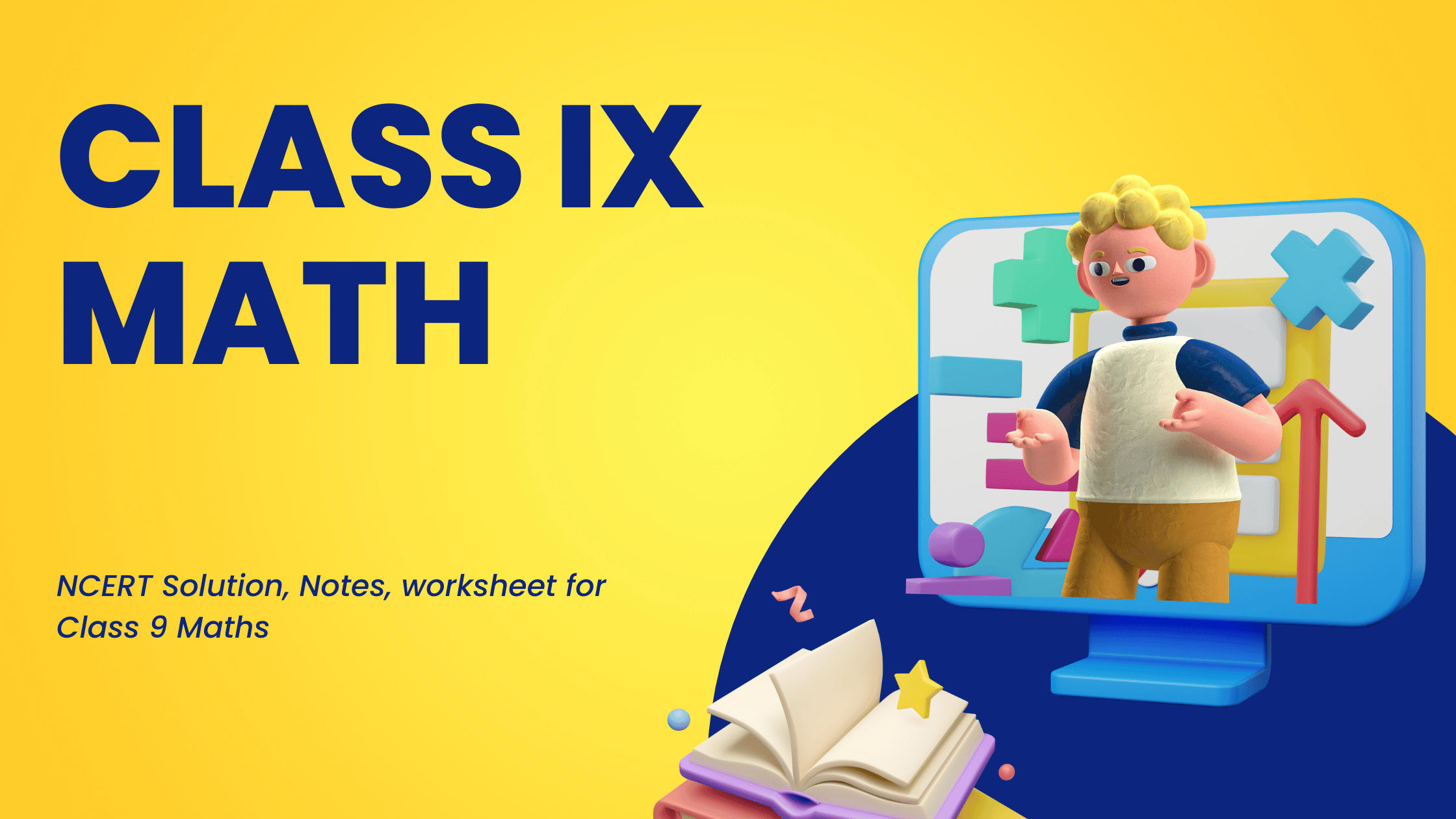# Class 9 Maths Study Material for CBSE, NCERT Solutions, Maths Worksheets

•• Welcome to our class 9 Maths page. In this page you can find class IX Maths Ncert solutions , notes, assignments, worksheets,Practice questions etc. You can share the link to free Class 9 NCERT Maths solutions with your classmates as all study material is free of cost.

• First read and understand the notes.
• Try to go through the solved examples of of the chapter you are reading
• Now it is the time to solve unsolved assignments and worksheets. You will find plenty of them here or on the respective chapter page.
All the best for better learning of your maths concepts. Hope we helped you when you were looking for class 9 Maths notes .

## Chapter 1:Number Systems

Notes & Worksheets
NCERT Solutions

## Chapter 2: Polynomials

Notes & Worksheets
NCERT Solutions

## Chapter 3: Coordinate Geometry

Notes & Worksheets
NCERT Solutions

## Chapter 4: Linear equations in two variables

Notes & Worksheets
NCERT Solutions

## Chapter 5: Introduction to Euclid Geometry

Notes & Worksheets
NCERT Solutions

## Chapter 6: Line and Angles

Notes & Worksheets
NCERT Solutions

## Chapter 7: Triangles

Notes & Worksheets
NCERT Solutions

Notes & Worksheets
NCERT Solutions

Worksheets
NCERT Solutions

## Chapter 10:Heron Formula

Notes & Worksheets
NCERT Solutions

## Chapter 11: Surface Area and Volume

Notes & Worksheets
NCERT Solutions

## Chapter 12: Statistics

Notes & Worksheets
NCERT Solutions

## Deleted Chapter 2023-2024

### Chapter Area of Parallelogram and Triangles

Notes & Worksheets
NCERT Solutions

### Chapter Constructions

Assignments/Worksheets

### Chapter Probability

Notes & Worksheets
NCERT Solutions

## Frequently asked Questions on CBSE Class 9 Maths

### How many chapters are there in Class 9 Maths?

There are total 12 chapters in Class 9 Maths as per the rationalized Syllabus. All the chapters will come in Board examination

### What is the best books for Class 9 Maths?

Couple of book
(a)NCERT Class 9 Science textbook
(b)NCERT Class 9 Science Exemplar
(c)RS Agarwal and RD Sharma

### Which is the easiest chapter of Class 9 Maths NCERT Text book?

Number system & Coordinate Geometry are the easiast chapter

### Is Class 9 Maths NCERT Text book Sufficient for CBSE Exams?

It is sufficient if you go through all the questions in the NCERT text book thoroughly and practice them all.However to enhance your Science skill , you can go for NCERT exemplar and other books

### what is the best way to learn the concept in Class 9 Maths?

First go through all the Notes and solved examples and Then do the exercises. Practice Lot of questions. The simple Mantra is Practice .Practice and Practice

### what is the deleted syllabus in Class 9 Maths?

Area of Parallelogram and Triangles:Full Chapter deleted
Constructions:Full Chapter deleted
Heron's Formula:Application of Heron’s Formula in finding the area of a quadrilateral.
Surface Area and Volumes:Surface areas and volumes of cubes, cuboids and right circular cylinders.
Probability:Full chapter deleted
Triangles:Inequalities in Triangle
Linear equation :Graphing of Linear equations in two Variable
Statistics: Introduction to Statistics:Collection of data, presentation of data — tabular form, ungrouped / grouped.Mean, median and mode of ungrouped data.